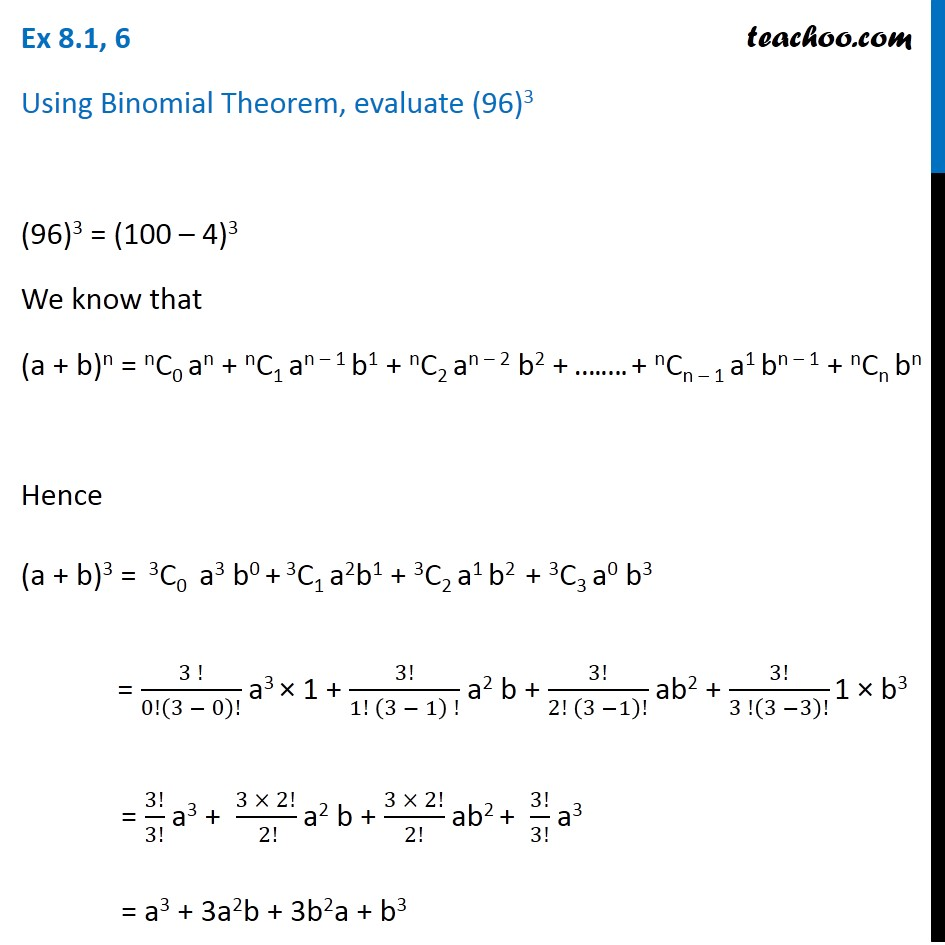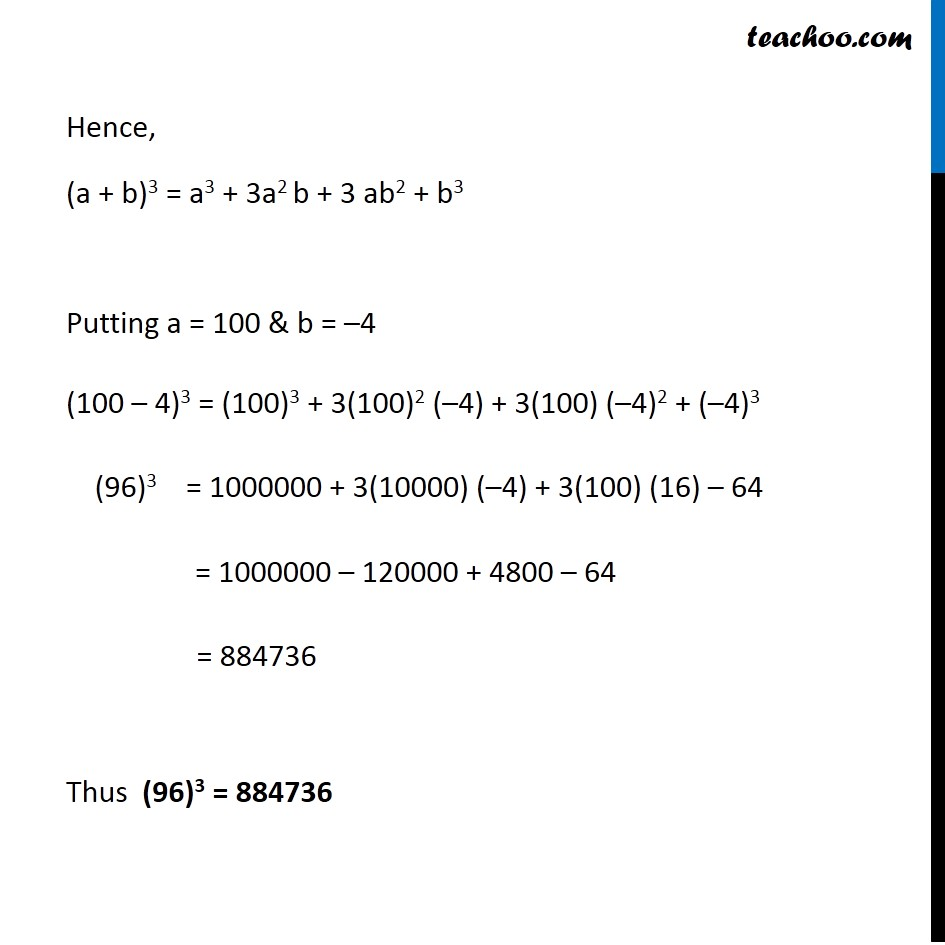Ex 7.1

Chapter 7 Class 11 Binomial Theorem
Serial order wiseLearn in your speed, with individual attention - Teachoo Maths 1-on-1 Class

### Transcript

Ex 7.1, 6 Using Binomial Theorem, evaluate (96)3 (96)3 = (100 – 4)3 We know that (a + b)n = nC0 an + nC1 an – 1 b1 + nC2 an – 2 b2 + ….…. + nCn – 1 a1 bn – 1 + nCn bn Hence (a + b)3 = 3C0 a3 b0 + 3C1 a2b1 + 3C2 a1 b2 + 3C3 a0 b3 = (3 !)/0!(3 − 0)! a3 × 1 + 3!/(1! (3 − 1) !) a2 b + 3!/(2! (3 −1)!) ab2 + 3!/(3 !(3 −3)!) 1 × b3 = 3!/3! a3 + (3 × 2!)/( 2!) a2 b + (3 × 2!)/2! ab2 + 3!/3! a3 = a3 + 3a2b + 3b2a + b3 Hence, (a + b)3 = a3 + 3a2 b + 3 ab2 + b3 Putting a = 100 & b = –4 (100 – 4)3 = (100)3 + 3(100)2 (–4) + 3(100) (–4)2 + (–4)3 (96)3 = 1000000 + 3(10000) (–4) + 3(100) (16) – 64 = 1000000 – 120000 + 4800 – 64 = 884736 Thus (96)3 = 884736